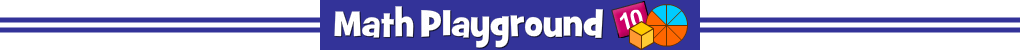Choose one or more numbers to subtract.
Can you make all 12 monster friends appear? And stay?
Math Monster Subtraction - Learning Connections
Essential Skills
Mental Math - practice subtracting numbers

Common Core Connection for Grades 1 and 2
Apply properties of operations as strategies to add and subtract.
Add and subtract within 20, demonstrating fluency for addition and subtraction within 10.
Add within 100, including adding a two-digit number and a one-digit number.
Fluently add and subtract within 20 using mental strategies.
More Math Games to Play